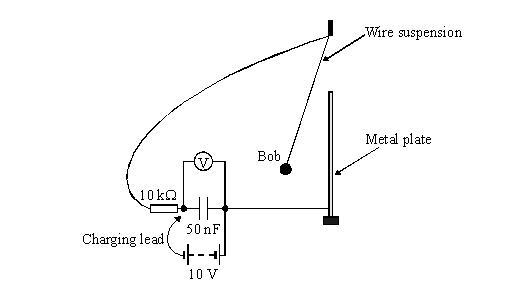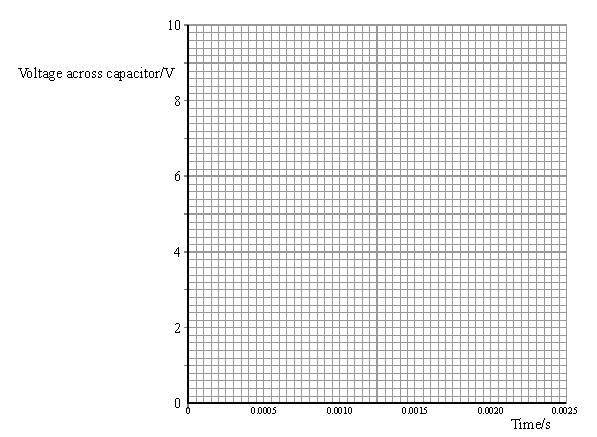# Investigating the contact times between a golf club and a golf ball

• Air

## Homework Statement

While investigating the contact times between a golf club and a golf ball, a student wanted to measure the contact times between metal objects – looking at how various factors (such as the material from which they were made, their mass, their speed on impact) affected the contact time.

The student devised a set-up in which a pendulum bob hit a plate. Initially it was thought that the laboratory millisecond timer could be used to measure contact times, but in a pilot experiment it was found that many of the times were less than a millisecond. The student decided to use the arrangement shown below, determining the contact times from readings of
the very high resistance voltmeter.a) The charging lead is used to charge the capacitor by briefly connecting it to the 10 V power supply. Calculate the charge stored in the capacitor.

b)Calculate the time constant t of this circuit when it is completed by contact of the pendulum bob with the plate.

c) How much charge will be left on each capacitor plate after a contact time equal to the circuit’s time constant t?

d) Hence calculate the voltmeter reading across the capacitor after contact time equal to t.

e) Calculate the voltage across the capacitor after contact times of 2t, 3t and 4t and plot your answers on the axes below.## Homework Equations

$Q = CV$,

$\tau = RC$,

$Q = \frac{Q_0}{e}$

## The Attempt at a Solution

a)
$Q = CV$
$= (50 \times 10^{-9}).(10) = 50 \times 10^{7} C$

b)
$\tau = RC$
$= (10 \times 10^3).(50 \times 10^{-9}) = 5 \times 10 ^{-4}s$

c)
$Q = \frac{Q_0}{e}$
$= \frac{5 \times 10^7}{e} = 1.8 \times 10^7 C$

d)
$v = \frac{Q}{C}$
$= \frac{1.8 \times 10^{-7}}{50 \times 10^-9} = 3.7V$

e)
This is where I am struggling. I don't know how to plot the values. To work out 2t, 3t and 4t, will I need to just times the voltage (3.7V) by 2, 3 and 4?

Thanks in advance.Hi Air,

For part e, you do not want to multiply the voltages by 2, 3, and 4. (That would increase the voltage, and you want the voltage to decrease.)

For this, I would use the time dependent formula for capacitor voltage for a discharging circuit:

$$V(t) = V_0 e^{-t/\tau}$$

where $\tau$ is the time constant.